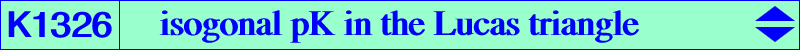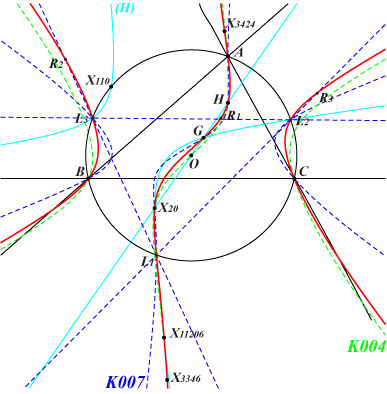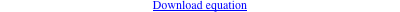too complicated to be written here. Click on the link to download a text file.X(2), X(4), X(20), X(3346), X(3424), X(11206), X(54032), X(54033) vertices of the Lucas triangle L1L2L3, see K007 vertices of the cevian triangle R1R2R3 of H in L1L2L3 infinite points of the altitudes of ABC Geometric properties :K1326 is the unique isogonal pK with respect to the Lucas triangle which is also a circum-cubic of ABC. It is the locus of M such that X(4), M and the isogonal conjugate of M with respect to the Lucas triangle are collinear. X(11206) is the isopivot i.e. the tangential of H. Hence, the tangents at H, L1, L2, L3 concur at X(11206). K1326 is pK(X6, X2979)'s sister as in Table 58. Note that X(2979) is the orthocenter of the Lucas triangle.